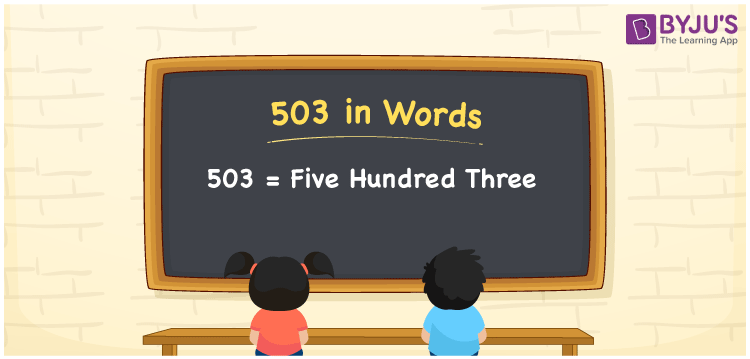# 503 in Words

503 in words can be written as Five Hundred Three. The concepts like counting numbers which are important from primary education are discussed here in brief. For instance, if you spend Rs. 503 in buying a doormat, then you can say that “I bought a door mat for Five Hundred Three Rupees”. The English alphabet is used to write 503 in words. Students can get a clear knowledge of numbers in words with the help of the study materials given at BYJU’S. The number 503 can be read as “Five Hundred Three” in English.

 503 in words Five Hundred Three Five Hundred Three in Numbers 503

## 503 in English Words## How to Write 503 in Words?

Place value chart and expanded form of 503 can be learnt easily in this section. There are three digits in the number 503. The place value chart for 503 is provided below for reference to help students understand the concepts effectively.

 Hundreds Tens Ones 5 0 3

The expanded form of 503 is provided below:

5 × Hundred + 0 × Ten + 3 × One

= 5 × 100 + 0 × 10 + 3 × 1

= 503

= Five Hundred Three

Hence, 503 in words is written as Five Hundred Three.

503 is a natural number that precedes 504 and succeeds 502.

503 in words – Five Hundred Three

Is 503 an odd number? – Yes

Is 503 an even number? – No

Is 503 a perfect square number? – No

Is 503 a perfect cube number? – No

Is 503 a prime number? – No

Is 503 a composite number? – Yes

## Frequently Asked Questions on 503 in Words

### How can 503 be written in words?

503 can be written as “Five Hundred Three” in words.

### Is 503 an odd number?

No, 503 is an odd number because it is not divisible by 2.

### Write Five Hundred Three in numbers.

Five Hundred Three can be written in numbers as 503.